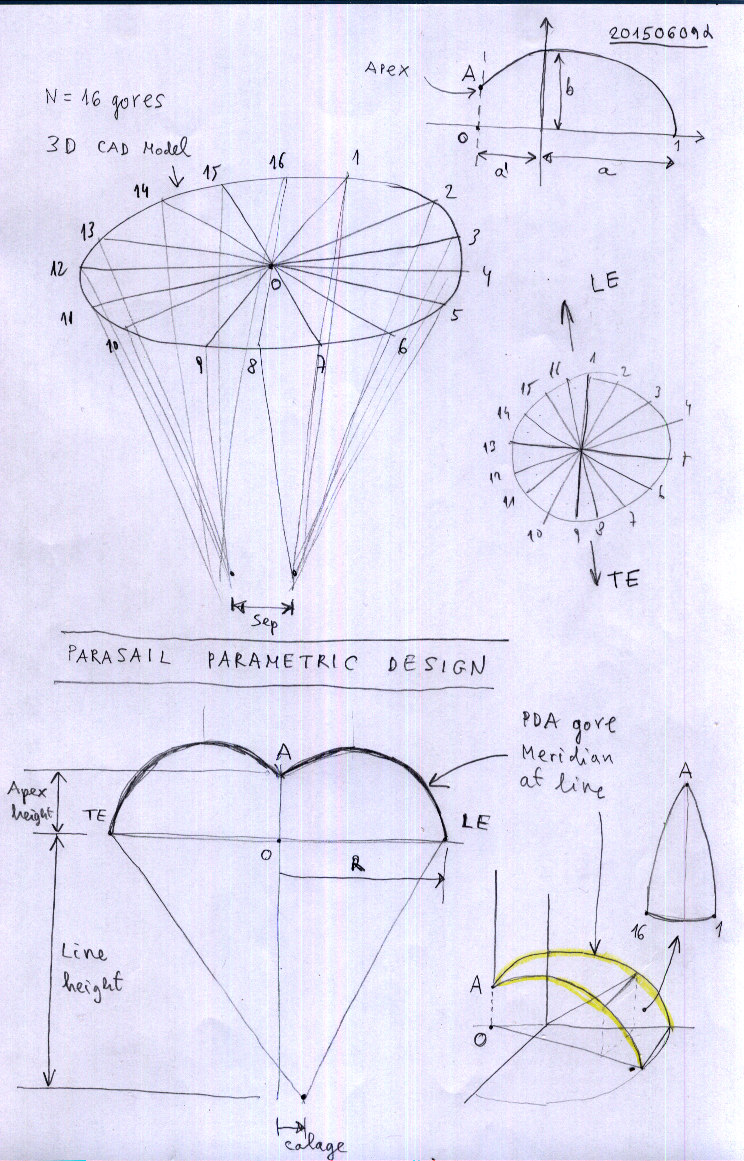SOLVING THE ROUND PARACHUTE-PARASAIL GORE

These notes will be implemented in the new laboratori program called "LEparachute"

Let's think about a round parachute. We can imagine the vault, like a "N" meridians equally spaced. The meridians can have different shapes, straight line (conical dome), parabolic, elliptical, elliptical amb pulled down apex..., and others. We call "gore" the piece of tissue between two successive meridians.  The problem to solve will be to find the flat development of the gore.

1. BASIC PARACHUTE-PARASAIL GEOMETRYFigure 1. Main geometry of the parachute-parasail

To define a simple geometry, let's define:

N= number of meridians = number of gores
A-TE = meridian line defining the parachute
R= radius of the dome (horizontal projection of the meridian)
O = center of the dome radius
Ah= Apex height
Lh= Line height
c = "calage", if the the parachute-parasail is asymmetrical

2. MERIDIAN DEFINITION2. Analytical equations for the meridian in a PDA parachute, using ellipse and parabolic modification. Many other shapes are possible to define.

These merdian line (yellow) will be defined using an ellipse and modification parabola as follows:
(using axes centered as in the figure)

Ellipse: x^2/a^2 + z^2/b^2 = 1  if x in [0,a]

or:   z= b · sqrt( 1 - x^2/a^2 )

if x in [-a,0]

z= b · sqrt( 1 - x^2/b^2 ) - k · x^2

where: k = (1/a'^2) · [b ·
sqrt( 1 - x^2/b^2 ) - c]

3. GORE SHAPE3. Gore projection in X-Y plan (left), and method for flat development (right)

Figure on the left is used to calculate the orange segment, which is the distance in a straight line between two points located at the same height in consecutive meridians.

Figure on the right contains basic instructions on how to calculate the gore. First, it is necessary to calculate the length along the meridian. Secondly, it is necessary to define a distance (green segment), which defines the width. The green distance (s), possibly deduced from the orange distance (d), using  a coefficient amplifier (ovalization)  to take into account the curvature of the panels. Using special laws of variation of "s" according to "d", it is possible to add special panels with vents, and other necessary devices...